# Pointer to Constant in C

## Pointer to Constant in C Language with Examples

In this article, I am going to discuss Pointer to Constant, Constant Pointer, and Constant Pointer to a Constant in C Language with Examples. Please read our previous articles, where we discussed Language with Examples. So, at the end of this article, you will understand the following pointers in detail.

1. What is Pointer to Constant?
2. What is a Constant Pointer?
3. Constant Pointer to a Constant
4. Difference between Pointer to Constant, Constant Pointer, and Constant Pointer to a Constant in C Language
##### What is Const in C?

The keyword const in C Programming Language can be applied to the declaration of any variable to specify that its value will not be changed. The most important point that you need to remember is the keyword const applies to whatever is there immediately to its left. If there is nothing to its left, it applies to whatever is there immediately to its right. With this kept in mind, let us proceed and understand the Pointer to Constant, Constant Pointer, and Constant Pointer to a Constant in C Language with Examples

##### Pointer to Constant in C Language:

The pointer will point to an address, where it cannot change the value at that address. But it is possible that this pointer can point to some other addresses. Assigning values is not possible in the pointer to constant. The pointer value can be incremented or decremented. The pointer is pointing to the constant data objects. If this is not clear at the moment, then don’t worry we will try to understand this concept with some examples.

Syntax: const <pointer-type> * <pointer-name>;
Example: const int*p;

##### Program to Understand Pointer to Constant in C Language

In the below example, we have created two integer variables i.e. a and b with the values 10 and 20 respectively. Then we created a pointer to the constant using the const keyword i.e. const int *p; Once we created the constant pointer, then we assign the address of the variable a to this pointer. As it is a pointer to constant, so we cannot change the address. So, when we are trying the change the value of the pointer i.e. *p = 5; it will give us an error. But it is possible to change the address of the pointer to a constant variable i.e. p = &b;.

```#include<stdio.h>
int main ()
{
int a = 10, b = 20;
const int *p;
p = &a;
printf ("value of a is %d, *p is %d \n", a, *p);
*p = 5;		//wrong you can not change value at address
printf ("value of a is %d, *p is %d \n", a, *p);
p = &b;		//you can change address
return 0;
}
```
###### Output: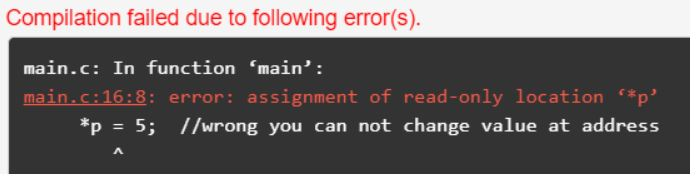##### Constant Pointer in C Language:

This concept is opposite to the Pointer to Constant concept. Here, the pointer will point to one address which we initialize at the declaration time. After that, we cannot change that address. But the value at that address we can change. We can assign a value to a constant pointer. Values cannot be incremented or decremented. Constant Pointer points to the data object.

Syntax: <pointer-type> * const <pointer-name> = <memory-address>;
Example: int*const p=&a;

##### Program to Understand Constant Pointer in C Language

In the below example, we have created two integer variables i.e. a and b with the values 10 and 20 respectively. Then we created a constant pointer using the const keyword i.e. int *const p = &a; and we also assign the address of the variable a at the time of this constant pointer declaration. As it is a pointer constant, so we cannot change the address. So, when we are trying the change the address of the pointer i.e.p = &b; it will give us an error. But it is possible to change the value of the constant pointer i.e. *p = 5;

```#include<stdio.h>
int main ()
{
int a = 10, b = 20;
int *const p = &a;
printf ("value of a is %d, *p is %d \n ", a, *p);
*p = 5;		//changing value at address
printf ("value of a is %d, *p is %d \n ", a, *p);
p = &b;		//changing address wrong
return 0;
}
```
###### Output: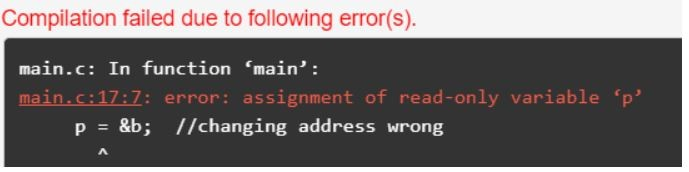Here, you can see we are getting the error “assignment of read-only variable ‘p’“. It means that the value of the variable ‘p’ which ‘p’ is holding cannot be changed. In the above code, we are changing the value of ‘p’ from &a to &b, which is not possible with constant pointers. Therefore, we can say that the constant pointer, which points to some variable, cannot point to another variable.

##### Constant Pointer to a Constant in C Language:

A constant pointer to a constant is a pointer in C Programming Language, which is a combination of the above two pointers i.e. Pointer to Constant and Constant Pointer. In this case, it is neither changing the address of the variable to which it is pointing nor changing the value placed at this address.
Syntax: const <type of pointer>* const <name of the pointer>;
Example: const int* const ptr;

Let us understand Constant Pointer to a Constant in C Language with an Example. In the below example, We have declared two variables, i.e., a and b with the values 10 and 20 respectively. Then we declare a constant pointer to a constant and then assign the address of the variable a. Then We try to change the value of the variable a through the pointer ptr as well as try to assign the address of variable b to this pointer ptr. Here, in both cases, we will get errors. And finally, we print the value of the variable, which is pointed by the pointer ‘ptr’.

```#include <stdio.h>
int main()
{
int a=10, b=20;
const int* const ptr=&a;
*ptr=12;  //wrong
ptr=&b;  //wrong
printf("Value of ptr is :%d",*ptr);
return 0;
}```
###### Output: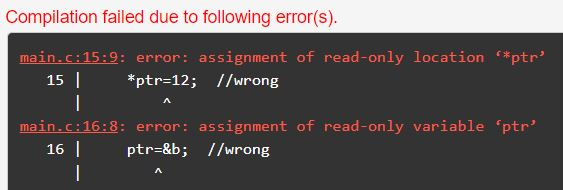#### Difference between Pointer to Constant, Constant Pointer, and Constant Pointer to a Constant

Let us understand the differences between Pointer to Constant (const int *ptr), Constant Pointer int * const ptr), and Constant Pointer to a Constant (const int * const ptr) in C Language.

##### Pointer to Constant (const int *ptr) :

This is a pointer to a constant integer. In this case, we cannot change the value which is pointed by the pointer ptr, but you can change the pointer itself i.e. you can change the address which is held by the pointer. So, here pointer value is constant but the address can be changed.

```#include<stdio.h>
#include<stdlib.h>
int main()
{
int a =10, b =20;
const int *ptr = &a;
//The following is illegal statement (assignment of read-only location *ptr)
//*ptr = b;
printf( "value pointed to by ptr: %d\n", *ptr);
// ptr address can be changed and is legal
ptr = &b;
printf( "value pointed to by ptr: %d\n", *ptr);
}```
###### Output: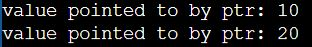There is no difference between const int *ptr and int const *ptr as both are pointers to a const integer and the position of *(asterisk) is also the same. So, the following program will also give you the same output as the previous one.

```int main()
{
int a =10, b =20;
int const *ptr = &a;
//The following is illegal statement (assignment of read-only location *ptr)
//*ptr = b;
printf( "value pointed to by ptr: %d\n", *ptr);
// ptr address can be changed and is legal
ptr = &b;
printf( "value pointed to by ptr: %d\n", *ptr);
}
```
##### Constant Pointer (int *const ptr):

This is a constant pointer to a non-constant integer. In this case, you cannot change the pointer ptr, but you can change the value pointed by the pointer ptr. So, here the pointer address is constant but the value can be changed.

```#include<stdio.h>
#include<stdlib.h>
int main()
{
int a =10, b =20;
int *const ptr = &a;
printf( "Value pointed to by ptr: %d\n", *ptr);
printf( "Address ptr is pointing to: %d\n", ptr);

//The following statement is illegal (assignment of read-only variable ptr)
//ptr = &b;

// changing the value at the address ptr is pointing to
*ptr = b;
printf( "Value pointed to by ptr: %d\n", *ptr);
printf( "Address ptr is pointing to: %d\n", ptr);
}
```
###### Output: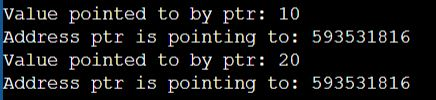Note: The Pointer always points to the same address, only the value at the location is changed.

##### Constant Pointer to a Constant (const int * const ptr):

This is a constant pointer to a constant integer. You can neither change the value pointed by ptr nor the address of the pointer ptr. So, here both pointer address and value are constant.

```#include<stdio.h>
#include<stdlib.h>
int main()
{
int a =10, b =20;
int char *const ptr = &a;

printf( "Value pointed to by ptr: %c\n", *ptr);
printf( "Address ptr is pointing to: %d\n\n", ptr);

//The following two statements are illegal
// ptr = &b; illegal statement (assignment of read-only variable ptr)
// *ptr = b; illegal statement (assignment of read-only location *ptr)

}```

Note: int const * const ptr is same as const int *const ptr.